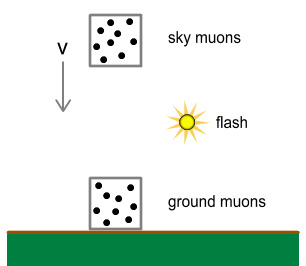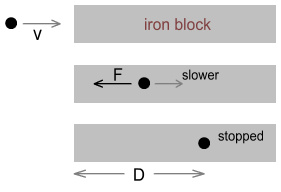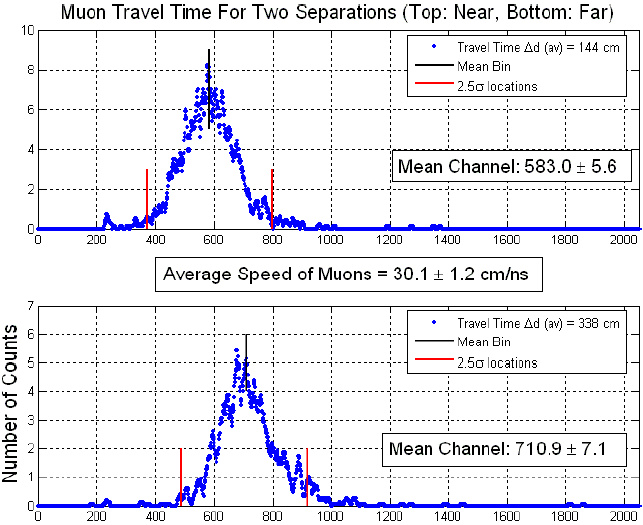Home

# Muons and Relativity

A muon is a type of subatomic particle that is created in particle collisions.  More properly called a “mu-meson”, it has a charge equal to that of an electron and a mass 206 times heavier.  As such it is often thought of as a heavy electron .

Unlike electrons however, muons are short lived and will quickly decay into other particles, typically an electron and some neutrinos.  Laboratory experiments show that their average life span (or rather half-life) is 2.2 microseconds.  That is, if we start with 1000 muons, after 2 microseconds we would expect around 500 to remain.  Then after a further 2 microseconds, 250 will remain, then 125, etc.

Muons are also created at high altitudes where the top of the atmosphere gets bombarded by solar and cosmic protons.  The generated muons rain down at high speed, some of them decaying partway down and others making it all the way to the ground at sea level.

According to our knowledge of decay times, we should expect the average distance they travel before decaying will depend on their initial speed.  For example if a muon were going at 50% of light speed, we’d expect it to travel (on average) 300 metres before decaying .

Based on this we should expect the number of muons detected at that 300m distance to be half of the original.  And at 600m down we'd expect a quarter of the original to remain, at 900m one eighth would remain, etc.  We can continue this down to sea level and determine what percentage would survive based on their original velocity.

Now it is noted that the number that make it to sea level is somewhat greater than what might be expected.  In fact the number that make it the ground is more than should be possible even if muons were assumed to be travelling at light speed (where the average decay distance calculates as 600m).

However, if we take into account the predictions of Special Relativity (SR) in which “moving clocks run slow”, we should expect muons moving at high velocity be time-dilated and thus decay more slowly.  In this way a muon would move a farther distance before terminating.

Most interesting though, is the actual counts of muons do indeed appear to match the amounts predicted by SR.  For example, muons going at 0.87c, where the Lorentz factor is 2, travel twice the distance before decaying.  And muons going at 0.995c, where the factor is 10, travel 10 times the distance before decaying.

This experiment would appear to be very good evidence for SR.  Because if we consider a muon to be a clock, it shows that its decay time is directly influenced by its speed relative to Earth and by the degree calculated according to SR.

But at the same time this explanation could also not be correct because speed is relative:  From the point of view of the atmospheric muon, it is standing still, and muons on the ground are moving.  And so the ground muons should be decaying more slowly instead.

This point should be immediately obvious but apparently it isn’t.  So it’s worth detailing the issue with a ‘thought experiment’ as follows.

Choose two locations; one at ground-level and the other at 2000ft.  There is an observer at both places, each with a convenient box in hand.  We place a flashbulb at the mid-point, i.e. at 1000ft.  The flashbulb is lit and gives off a brief pulse.

When the observer at the 2000ft altitude sees the flash, he quickly places the box around a group of a hundred muons that just happen to be passing by and in the downward direction at exactly 0.995c.  The box is given a quick downward push so that it matches the 0.995c velocity and heads down with the muons, without making contact with any of them.

When the observer at the ground-level altitude sees the flash, he quickly places the box around a group of a hundred muons that he finds sitting stationary just above the ground (assume these muons aren’t affected by gravity).  The box and muons then stay at that level without moving.A box containing muons coming down from the sky is approaching a similar box on the ground.  An equal number of muons were boxed at the same time.  The flashbulb ensures both groups were boxed simultaneously (this is also an accepted method in SR of synchronizing two clocks at a distance ).

The muons boxed at 2000ft were going at 0.995c.  So according to SR, they will decay 10 times more slowly than the (stationary) ones at ground-level.  Therefore when the boxes meet side-by-side at ground-level, we should expect there to be more muons in the “sky box” than in the “ground box”.

But according to the muons boxed at 2000ft, they are motionless and the muons at ground-level (along with the Earth) are rushing upward at 0.995c.  From their perspective, muons at ground-level will be decaying 10 times slower.  Therefore when the boxes meet side-by-side, we should expect there to be more muons in the ground-box than in the sky-box.

Clearly this is a contradiction as each box is required to contain more muons than the other!  To make this more explicit, suppose that shortly after the muons are boxed, everything else ceases to exist.  The universe now consists solely of two identical boxes containing 100 muons heading toward each other.  When they meet, which box will contain fewer muons?  On what grounds do we favour one over the other?  Placing the Earth back into the picture should not change anything SR-wise, and so the question remains the same.

Therefore in order to solve the question of why the “moving muons” outlive the “stationary muons”, it will be necessary to explore other possibilities.

One possibility is that the muons coming down through the atmosphere are not going just under light speed, but somewhat faster.  In this way the muons will travel a greater distance before decaying.  E.g. a muon going at double light speed will travel 1200m before decaying, a muon going at 10c will go 6000m, etc.

This possibility would likely strike the relativity supporter as absurd.  So much so, that they would probably not even consider it in the first place.  One reason for this has to do with conditioned thinking.  Another (more scientific) reason though is because the speed of the muons has already been measured and seen to be less than light.  But is that really the case?

To understand this, it is recommended to watch this video showing the famous 1962 “Frisch & Smith” experiment on muon (mu-meson) decay.  It is rather long but quite well presented:

In summary, muons are created at the top of the atmosphere and come down at a range of speeds; some slow, some fast.  What the experimenters need to do is ‘filter out’ the slow muons so that only the very fast ones are measured.  The way they do this is to put a large block of iron above the detector.  This slows down the muons.  The slow ones will come to a stop and decay inside the iron block before reaching the detector.  Only fast muons will make it to the other side and get detected.  The thickness of the iron block will determine the minimum speed of what gets through.  In the experiment it was built at 2.5 feet thick, and this apparently let through only muons that were originally going at 0.9950c (99.5% the speed of light) or faster.

The muons that make it through the block it then pass through a cylinder of clear plastic called a ‘scintillator’.  The purpose of the scintillator is not just to detect the particles but also to filter out anything faster than a certain speed.  The thickness/depth of the scintillator determines the upper speed limit.  In this case they set it so that the original upper speed (prior to reaching the iron block) is 0.9954c.  Anything beyond that passes through the scintillator before it can decay and give off a flash of light that they can observe.  So what we end up looking at are muons in the range of 0.9950c to 0.9954c.

Now it is true to say that, once in the scintillator we can measure the muon’s speed because we have a distance and a time.  But keep in mind that the muons at this point have been slowed down from their original assumed speed of 0.995c and are now going much slower.  Based on the fact that some of them decay within the cylinder (in 2 microseconds) and the cylinder looks 60cm deep means they must now be going 300,000m/s, or 1000 times slower than light at that point.  So we can’t use the scintillator to measure the original speed, only the slowed-down speed.

So the real question is, how did they determine the original 0.995c speed?  They describe this at 22:00 but don’t give enough detail.  So I’ll try do so here.

As charged particles (such as muons) move through the iron they experience a drag force.  To simplify this, let us suppose that the force is of known fixed strength while the muon is in motion, and stops when the muon stops.  The following diagrams describe the situation:• In the top frame, a muon is coming from the left at velocity v and is about to enter the iron.
• In the middle frame, the muon is passing through the iron and experiencing a steady backward force of F.  Because of this, its velocity steadily decreases.
• In the bottom frame, the muon has come to a halt after travelling distance D.

So how do we determine how far it travels before stopping?  This can be done using energy conservation equations.

The muon strikes the iron with a certain amount of kinetic energy.  As it passes through, this energy gets steadily converted to potential energy according to the equation:

Energy(potential) = force * distance

If the muon comes to a stop, this means the original kinetic energy of the muon has been fully converted.  As you probably know, kinetic energy is given by the equation:

E(kinetic) = ˝ mass * velocity2

By setting these energy equations equal we can determine the stopping distance from a known initial velocity.  Alternatively, if we know the stopping distance, we can instead determine the initial velocity.  I.e. we can determine how fast the muon was going when it hit the iron.  And this is what we are trying to figure out in this situation.

The ˝ m v2 equation however is for classical mechanics.  There is another equation that is used in relativity.  It is of the form:

E(kinetic) = Erelativistic – Erest

= E – E0  =  m*c2 – m0*c2

Where m0 is the rest mass and m is the relativistic mass.  m is equal to m0/sqrt(1-v2/c2), i.e. the rest mass multiplied by the Lorentz Transform.

What is the difference between these two functions?  For low velocities there is basically no difference.  But for high velocities, the velocity calculated by the classical formula can allow v>c.  Whereas the relativistic formula ensures v<c.

The above is simplified.  In reality the force experienced won’t be constant but a complicated function of velocity.  The actual function used is called the Bethe Formula.  A summary is here:

en.wikipedia.org/wiki/Bethe_formula

Notice there are two versions: a non-relativistic one for low velocities (eqn. 2), and a relativistic one for high velocities (eqn. 1).  The relativistic one contains the Lorentz Transform.

So which equation do you think they use?  If you are a believer in relativity you will use the relativistic equation, correct?  Otherwise you will get a result that is inconsistent with relativity, and therefore not considered a proper test of relativity.

But by using the relativistic energy equation you get different results for v.  And you guarantee v<c.  Whereas using the classical equation can give v>c, especially for high-energy situations.  But this possibility is immediately rejected because everyone “knows” it’s not possible.  In fact, it is usually not even considered.

So the experiments for muon decay unknowingly end up with the sqrt(1-v2/c2) function built into the outcome.  And thus, no surprise, “confirms” the function to be true!

#### Direct measurement of speed

Muon speeds can also be directly measured by timing how long it takes them to pass between two locations.  Experiments that employ this method report that the maximum speed recorded is always less than light.  On the face of it this would appear good evidence that muons, and presumably other particles, are restricted by a light-speed limit.

A closer inspection of the experiments however reveals that what is being reported is not the maximum speed of individual muons but the average speed of many.

Take this reference for example .  It’s an experiment that measures how quickly muons move between two flat horizontal detector paddles located one above the other.

The below charts were reproduced from Figure 5.  It shows the results of multiple recorded speeds.If we look at the top chart, it shows the average occurring around channel 583, which corresponds to 30.1cm/ns and is close to the speed of light (30cm/ns).  But the range shown within the red lines (within 2.5 standard deviations) goes from 380 to 800.  That would suggest a speed range from 583/800c= 0.73c  to  583/380c= 1.53c

Actually the lowest channel where an event occurs appears to be 220, and this translates to 583/220c= 2.7c.

If we look at the bottom chart, the average is 710.9 and the lowest channel is about 190.  That translates to 710.9/190c= 3.7c

Furthermore, when they quote the average speed they show it with +/-, e.g. 29.8+/-2.5 cm/ns.  This gives the impression that the speeds range from 29.8-2.5 to 29.8+2.5 cm/ns.  In reality that +/- is not a range but an estimated error of the average speed calculation – the range in speeds is much broader.

Let’s be clear.  If the average speed of many particles is c, and some are less than c, this requires that others must be moving faster than c.  There’s no way around this, unless we redefine what an ‘average’ is.

So why do they highlight the average speed and not maximum and minimum speeds?  Hard to know, but perhaps because a maximum >c is considered impossible and therefore shouldn’t be reported on.

#### Time dilation or Length contraction?

Returning to the Frisch & Smith video, there is something else of interest.  In the video, time dilation calculations are done to explain why muons coming down through the atmosphere are decaying slowly and why they outlive stationary muons at ground level.  But as pointed out earlier, from the view of the muons coming down, they are motionless and the ground-muons are moving up at exactly the same speed.  So why not do the calculations the other way around?

Toward the end (from 31:30) the presenters raise this question.  Their explanation is that from the view of the muons coming down, length contraction is occurring and the ground appears much closer.  Therefore they travel a shorter distance and reach the ground before decaying.  This explanation is utterly inconsistent because the same amount of length contraction must also be happening from the view of the ground-muons, allowing them to also travel a shorter distance toward the muons coming down and reach them before decaying.

In short, they use time dilation from one direction and length contraction from the other!  It’s hard to say whether the presenters are aware of their inconsistent logic or if they are just reciting it with a straight face.  But it seems they believe it.

  There are also less-common positive-charged muons of equal mass, making them “heavy positrons”.
  Calculation: 0.5 * 3x108 * 2x10-6 = 300m
  T.M. Helliwell, Special Relativity, Chapter 6.2, option 4
  http://web.mit.edu/lululiu/Public/pixx/not-pixx/muons.pdf

 Previous Contents Next chapter